Скачать презентацию Implementation and performance evaluation of LT and Raptor

• Количество слайдов: 37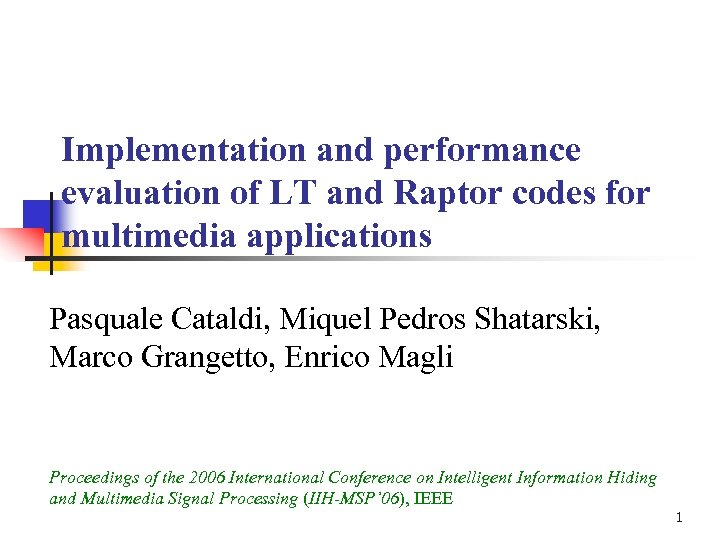Implementation and performance evaluation of LT and Raptor codes for multimedia applications Pasquale Cataldi, Miquel Pedros Shatarski, Marco Grangetto, Enrico Magli Proceedings of the 2006 International Conference on Intelligent Information Hiding and Multimedia Signal Processing (IIH-MSP’ 06), IEEE 1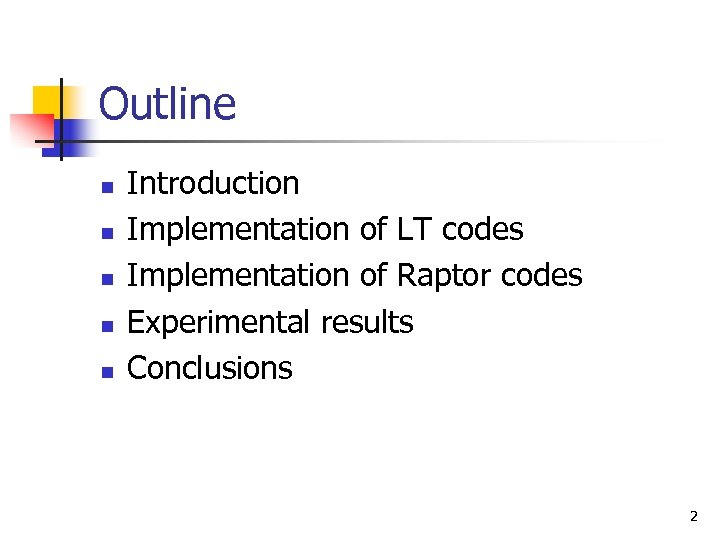Outline n n n Introduction Implementation of LT codes Implementation of Raptor codes Experimental results Conclusions 2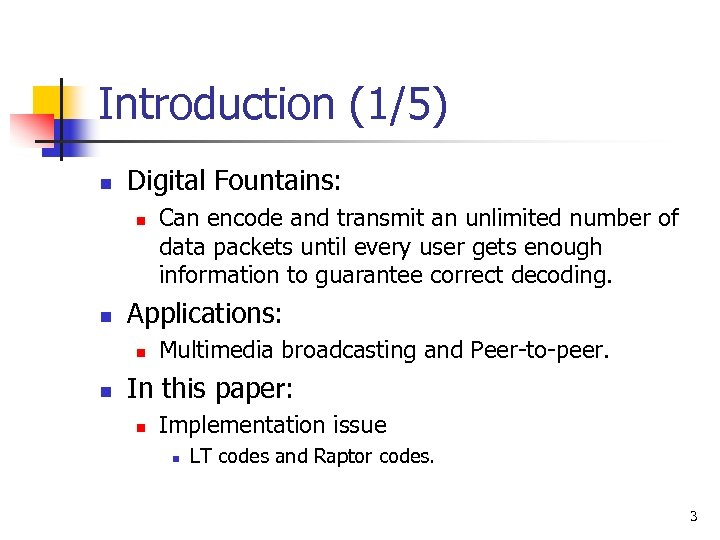Introduction (1/5) n Digital Fountains: n n Applications: n n Can encode and transmit an unlimited number of data packets until every user gets enough information to guarantee correct decoding. Multimedia broadcasting and Peer-to-peer. In this paper: n Implementation issue n LT codes and Raptor codes. 3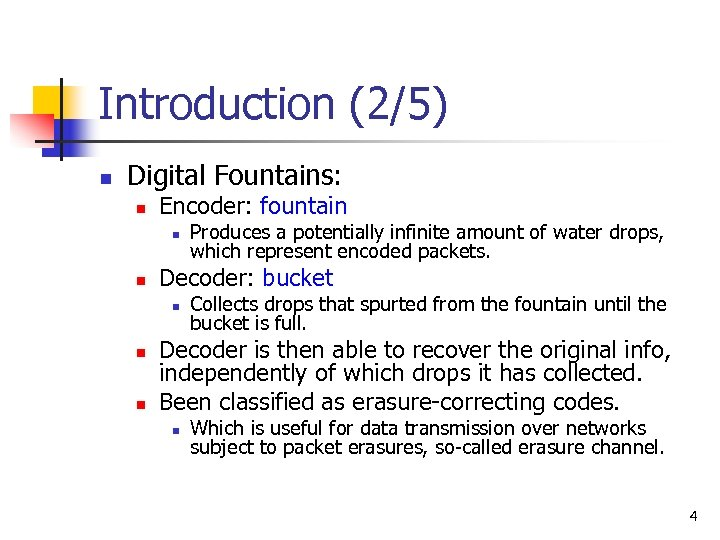Introduction (2/5) n Digital Fountains: n Encoder: fountain n n Decoder: bucket n n n Produces a potentially infinite amount of water drops, which represent encoded packets. Collects drops that spurted from the fountain until the bucket is full. Decoder is then able to recover the original info, independently of which drops it has collected. Been classified as erasure-correcting codes. n Which is useful for data transmission over networks subject to packet erasures, so-called erasure channel. 4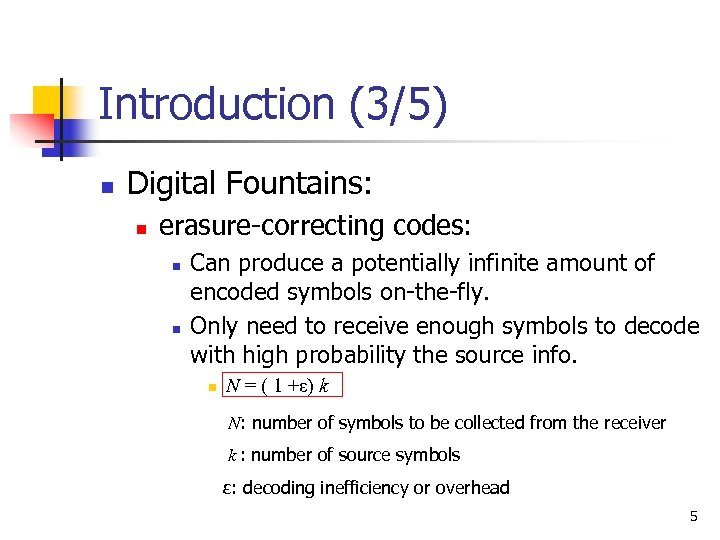Introduction (3/5) n Digital Fountains: n erasure-correcting codes: n n Can produce a potentially infinite amount of encoded symbols on-the-fly. Only need to receive enough symbols to decode with high probability the source info. n N = ( 1 +ε) k N: number of symbols to be collected from the receiver k : number of source symbols ε: decoding inefficiency or overhead 5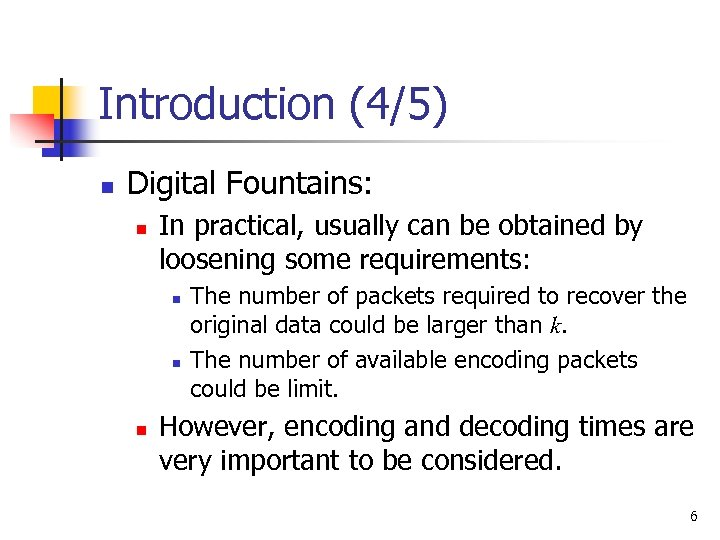Introduction (4/5) n Digital Fountains: n In practical, usually can be obtained by loosening some requirements: n n n The number of packets required to recover the original data could be larger than k. The number of available encoding packets could be limit. However, encoding and decoding times are very important to be considered. 6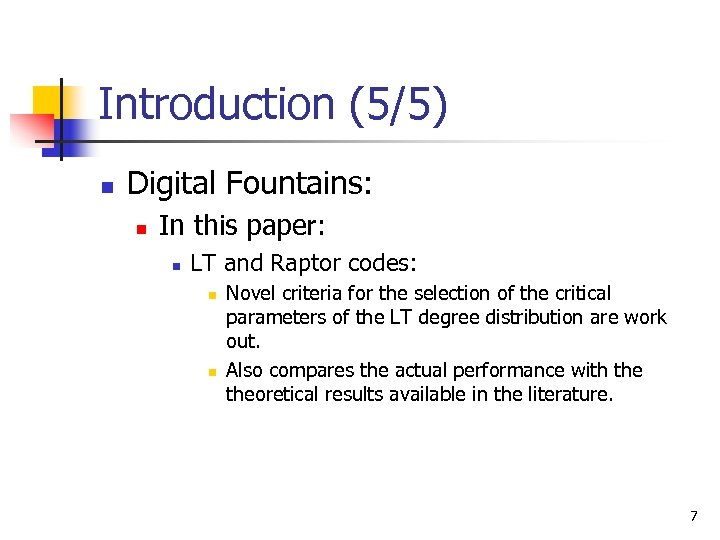Introduction (5/5) n Digital Fountains: n In this paper: n LT and Raptor codes: n n Novel criteria for the selection of the critical parameters of the LT degree distribution are work out. Also compares the actual performance with theoretical results available in the literature. 7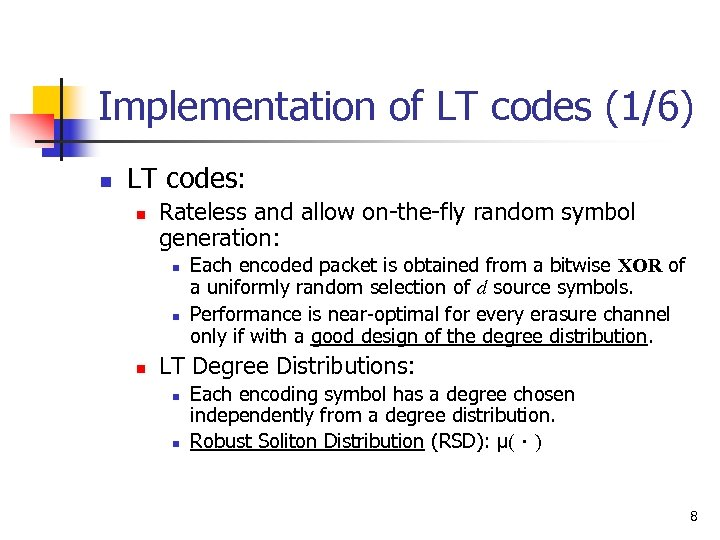Implementation of LT codes (1/6) n LT codes: n Rateless and allow on-the-fly random symbol generation: n n n Each encoded packet is obtained from a bitwise XOR of a uniformly random selection of d source symbols. Performance is near-optimal for every erasure channel only if with a good design of the degree distribution. LT Degree Distributions: n n Each encoding symbol has a degree chosen independently from a degree distribution. Robust Soliton Distribution (RSD): μ(．) 8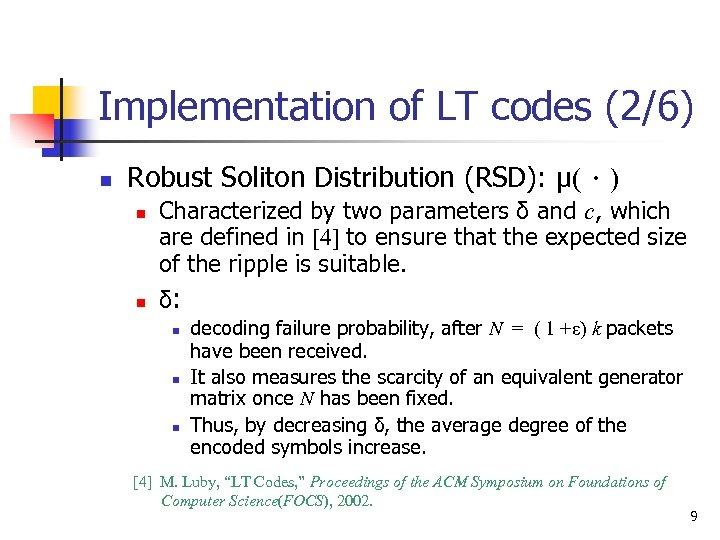Implementation of LT codes (2/6) n Robust Soliton Distribution (RSD): μ(．) n n Characterized by two parameters δ and c, which are defined in  to ensure that the expected size of the ripple is suitable. δ: n n n decoding failure probability, after N = ( 1 +ε) k packets have been received. It also measures the scarcity of an equivalent generator matrix once N has been fixed. Thus, by decreasing δ, the average degree of the encoded symbols increase.  M. Luby, “LT Codes, ” Proceedings of the ACM Symposium on Foundations of Computer Science(FOCS), 2002. 9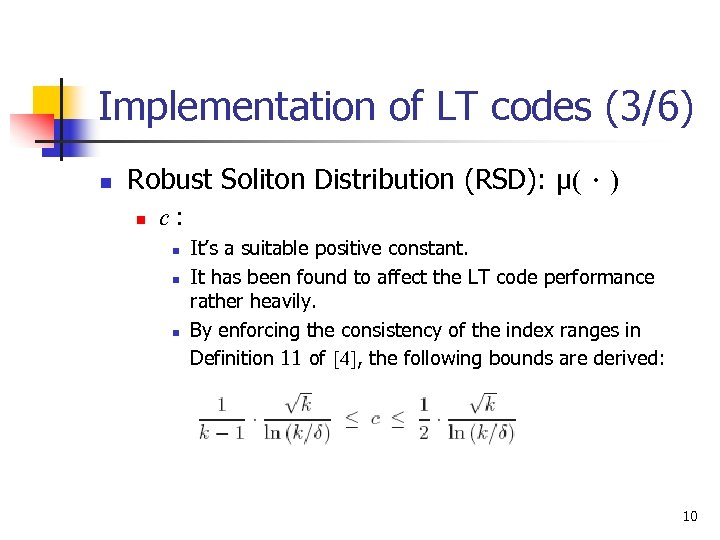Implementation of LT codes (3/6) n Robust Soliton Distribution (RSD): μ(．) n c: n n n It’s a suitable positive constant. It has been found to affect the LT code performance rather heavily. By enforcing the consistency of the index ranges in Definition 11 of , the following bounds are derived: 10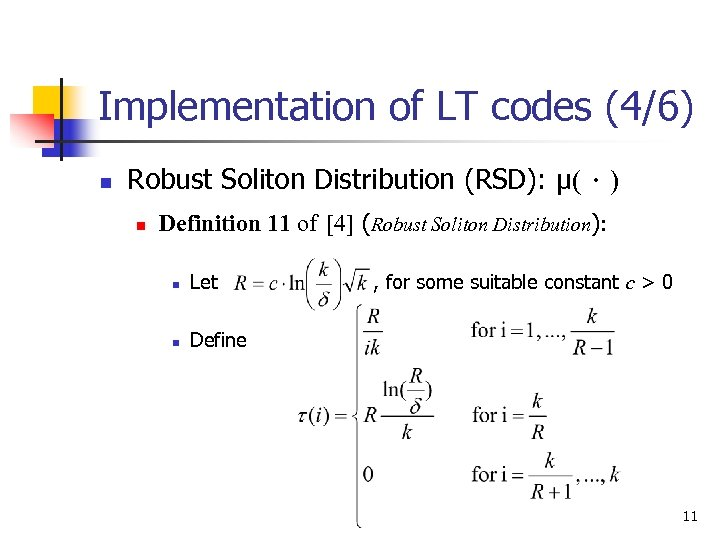Implementation of LT codes (4/6) n Robust Soliton Distribution (RSD): μ(．) n Definition 11 of  (Robust Soliton Distribution): n Let n , for some suitable constant c > 0 Define 11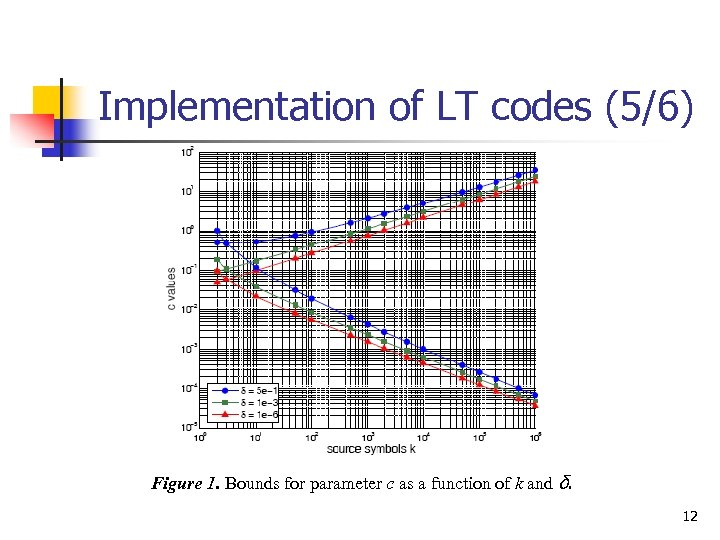Implementation of LT codes (5/6) Figure 1. Bounds for parameter c as a function of k and δ. 12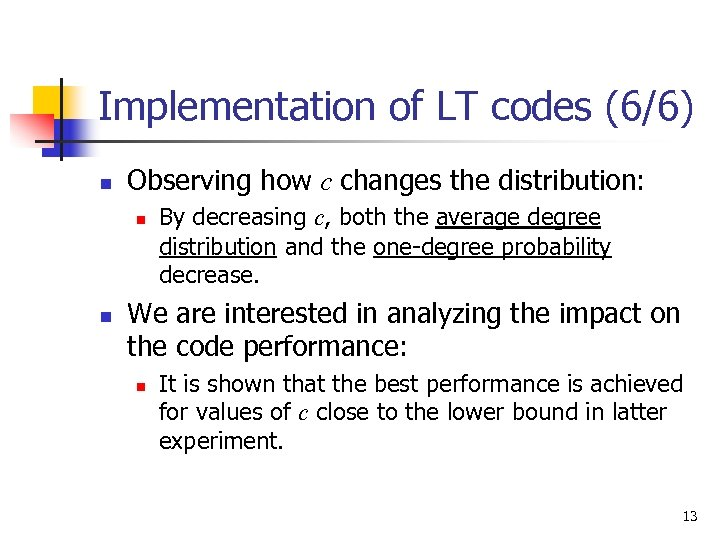Implementation of LT codes (6/6) n Observing how c changes the distribution: n n By decreasing c, both the average degree distribution and the one-degree probability decrease. We are interested in analyzing the impact on the code performance: n It is shown that the best performance is achieved for values of c close to the lower bound in latter experiment. 13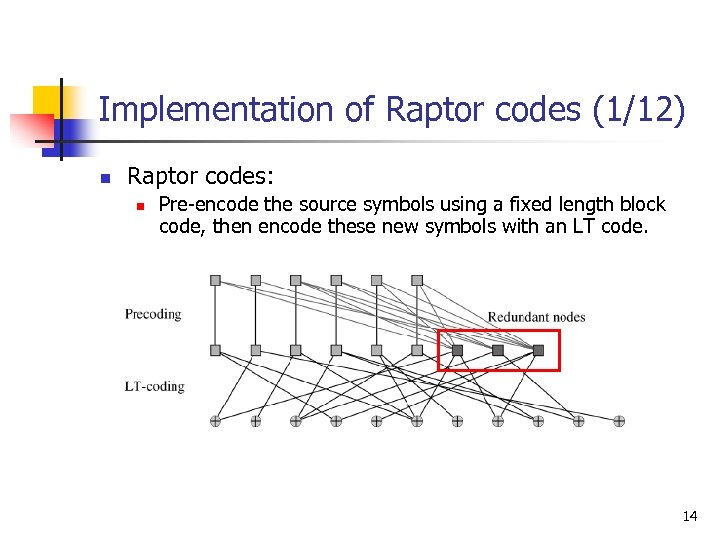Implementation of Raptor codes (1/12) n Raptor codes: n Pre-encode the source symbols using a fixed length block code, then encode these new symbols with an LT code. 14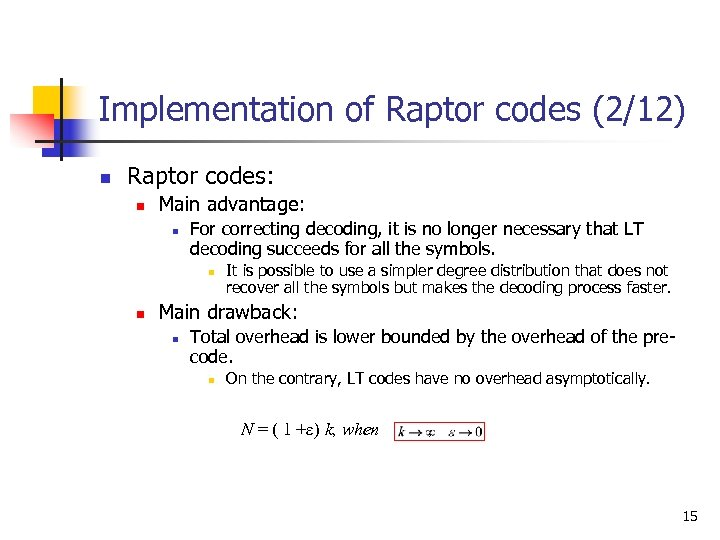Implementation of Raptor codes (2/12) n Raptor codes: n Main advantage: n For correcting decoding, it is no longer necessary that LT decoding succeeds for all the symbols. n n It is possible to use a simpler degree distribution that does not recover all the symbols but makes the decoding process faster. Main drawback: n Total overhead is lower bounded by the overhead of the precode. n On the contrary, LT codes have no overhead asymptotically. N = ( 1 +ε) k, when , 15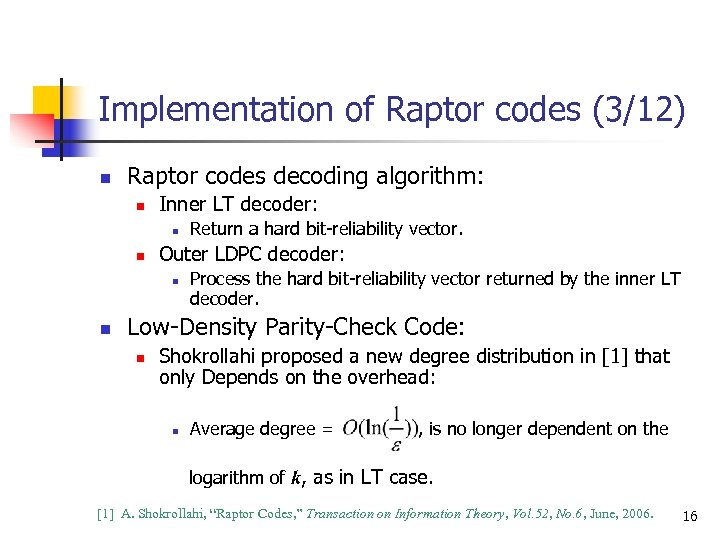Implementation of Raptor codes (3/12) n Raptor codes decoding algorithm: n Inner LT decoder: n n Outer LDPC decoder: n n Return a hard bit-reliability vector. Process the hard bit-reliability vector returned by the inner LT decoder. Low-Density Parity-Check Code: n Shokrollahi proposed a new degree distribution in  that only Depends on the overhead: n Average degree = , is no longer dependent on the logarithm of k, as in LT case.  A. Shokrollahi, “Raptor Codes, ” Transaction on Information Theory, Vol. 52, No. 6, June, 2006. 16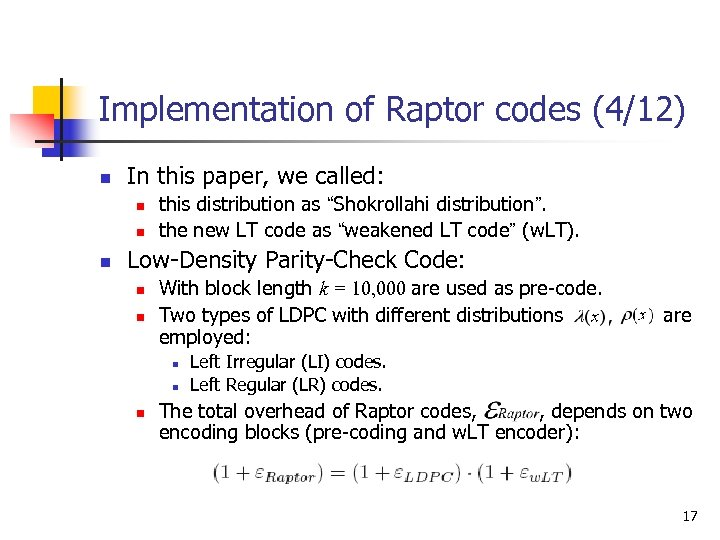Implementation of Raptor codes (4/12) n In this paper, we called: n n n this distribution as “Shokrollahi distribution”. the new LT code as “weakened LT code” (w. LT). Low-Density Parity-Check Code: n n With block length k = 10, 000 are used as pre-code. Two types of LDPC with different distributions employed: n n n are Left Irregular (LI) codes. Left Regular (LR) codes. The total overhead of Raptor codes, , depends on two encoding blocks (pre-coding and w. LT encoder): 17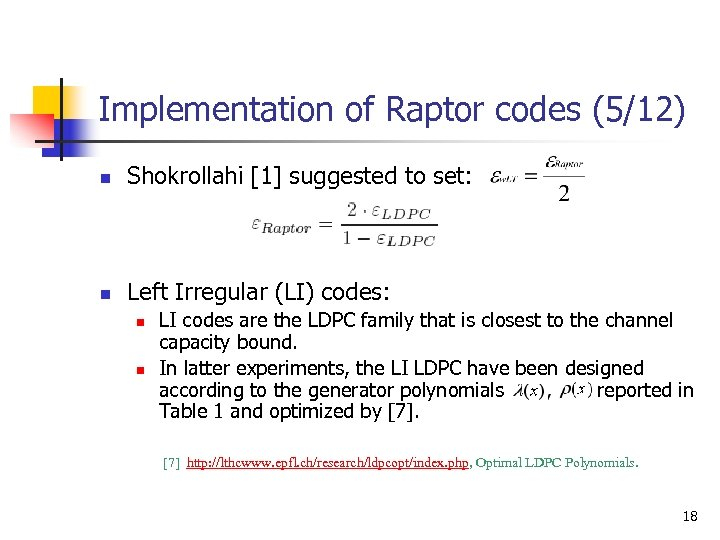Implementation of Raptor codes (5/12) n Shokrollahi  suggested to set: n Left Irregular (LI) codes: n n LI codes are the LDPC family that is closest to the channel capacity bound. In latter experiments, the LI LDPC have been designed according to the generator polynomials reported in Table 1 and optimized by  http: //lthcwww. epfl. ch/research/ldpcopt/index. php, Optimal LDPC Polynomials. 18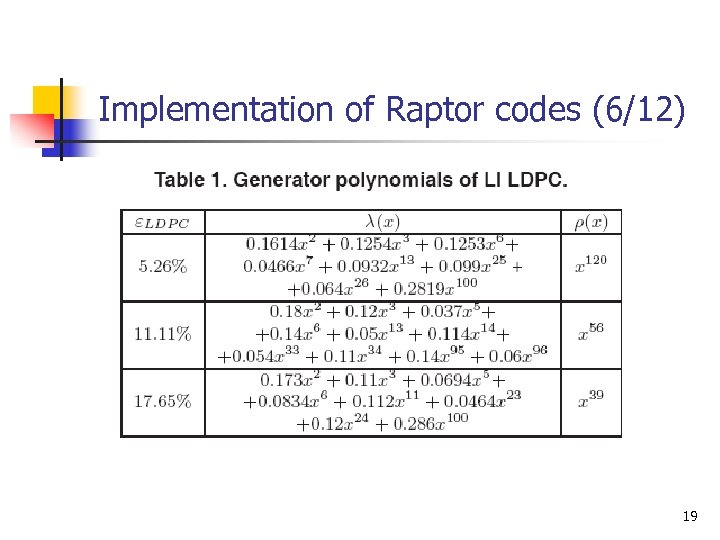Implementation of Raptor codes (6/12) 19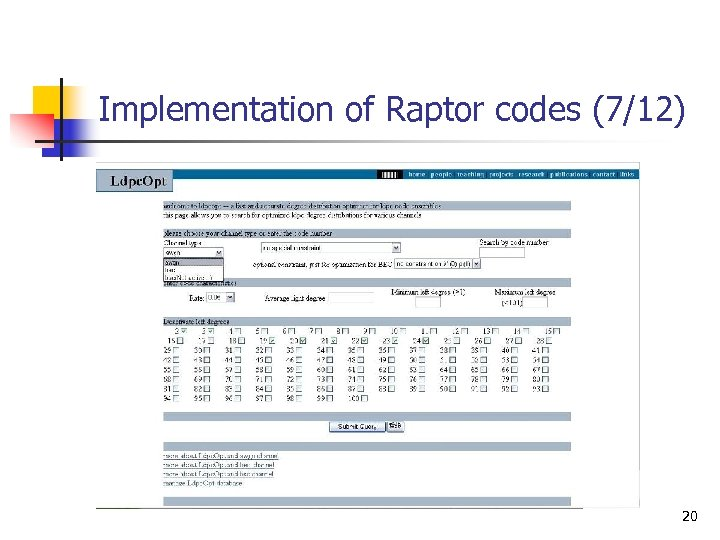Implementation of Raptor codes (7/12) 20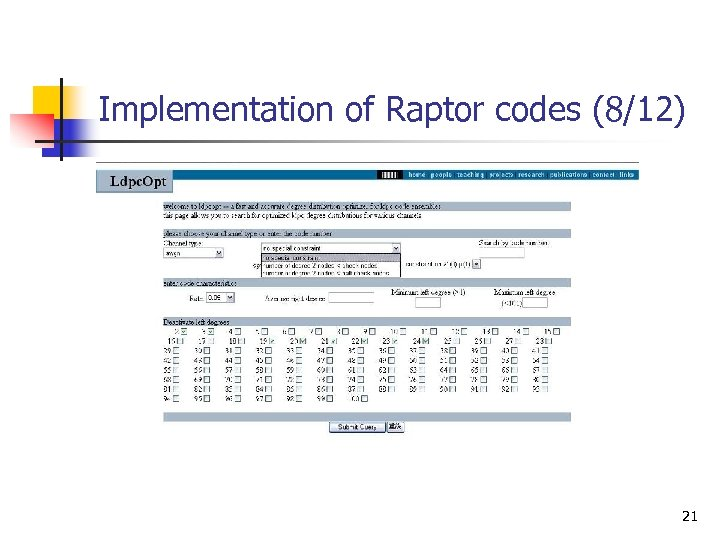Implementation of Raptor codes (8/12) 21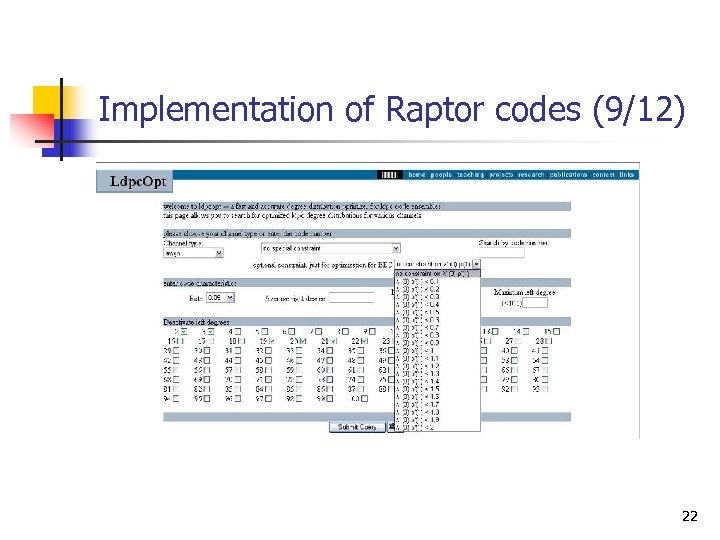Implementation of Raptor codes (9/12) 22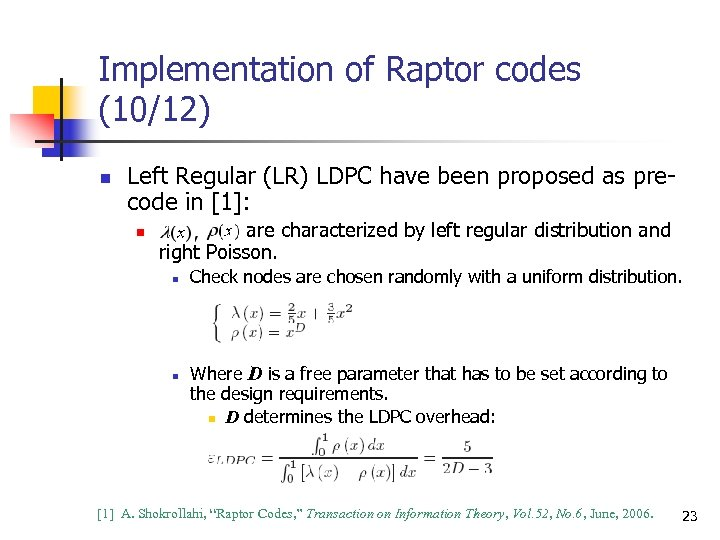Implementation of Raptor codes (10/12) n Left Regular (LR) LDPC have been proposed as precode in : n are characterized by left regular distribution and right Poisson. n n Check nodes are chosen randomly with a uniform distribution. Where D is a free parameter that has to be set according to the design requirements. n D determines the LDPC overhead:  A. Shokrollahi, “Raptor Codes, ” Transaction on Information Theory, Vol. 52, No. 6, June, 2006. 23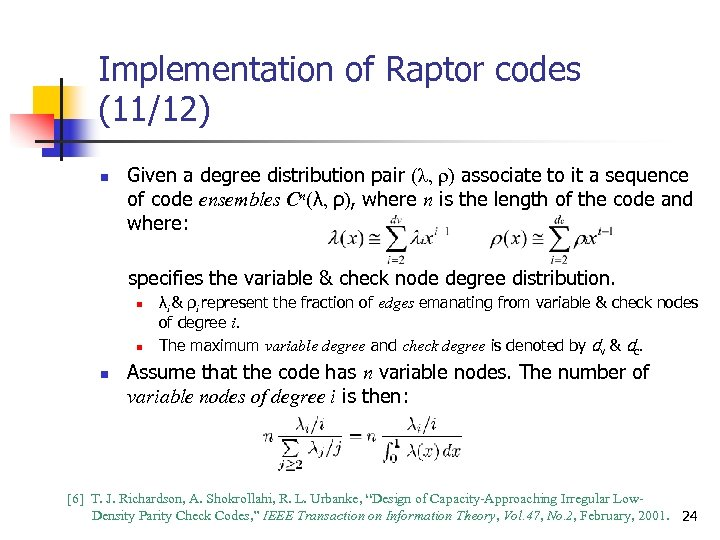Implementation of Raptor codes (11/12) n Given a degree distribution pair (λ, ρ) associate to it a sequence of code ensembles Cn(λ, ρ), where n is the length of the code and where: specifies the variable & check node degree distribution. n n n λi & ρi represent the fraction of edges emanating from variable & check nodes of degree i. The maximum variable degree and check degree is denoted by dv & dc. Assume that the code has n variable nodes. The number of variable nodes of degree i is then:  T. J. Richardson, A. Shokrollahi, R. L. Urbanke, “Design of Capacity-Approaching Irregular Low. Density Parity Check Codes, ” IEEE Transaction on Information Theory, Vol. 47, No. 2, February, 2001. 24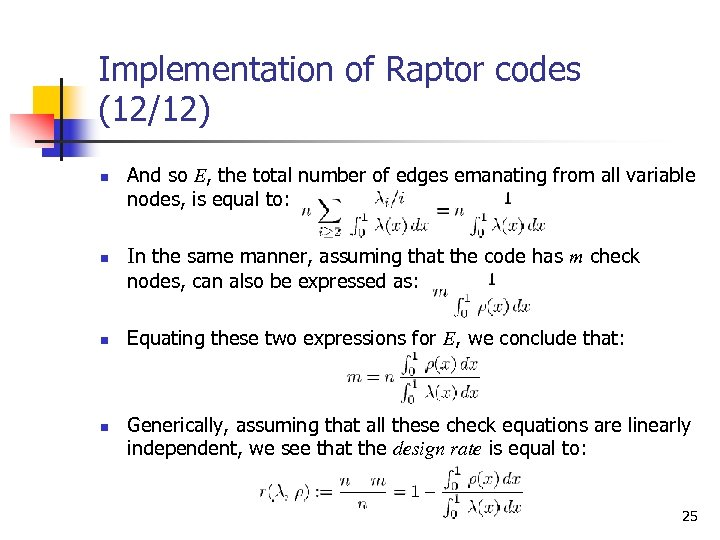Implementation of Raptor codes (12/12) n n And so E, the total number of edges emanating from all variable nodes, is equal to: In the same manner, assuming that the code has m check nodes, can also be expressed as: Equating these two expressions for E, we conclude that: Generically, assuming that all these check equations are linearly independent, we see that the design rate is equal to: 25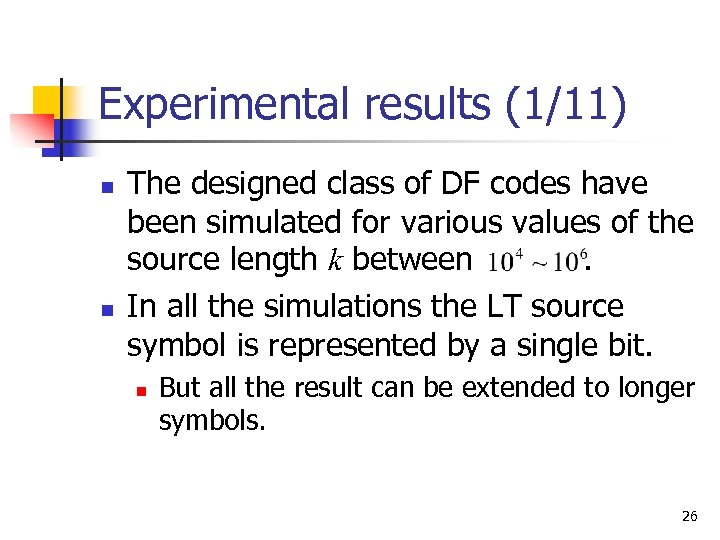Experimental results (1/11) n n The designed class of DF codes have been simulated for various values of the source length k between. In all the simulations the LT source symbol is represented by a single bit. n But all the result can be extended to longer symbols. 26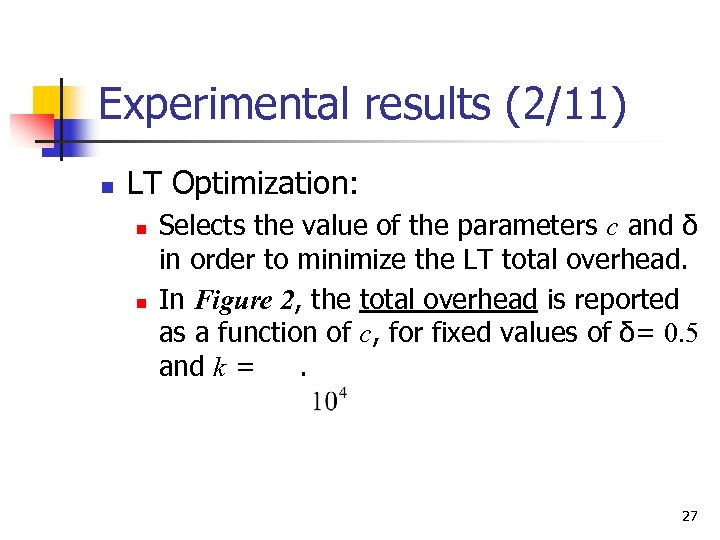Experimental results (2/11) n LT Optimization: n n Selects the value of the parameters c and δ in order to minimize the LT total overhead. In Figure 2, the total overhead is reported as a function of c, for fixed values of δ= 0. 5 and k =. 27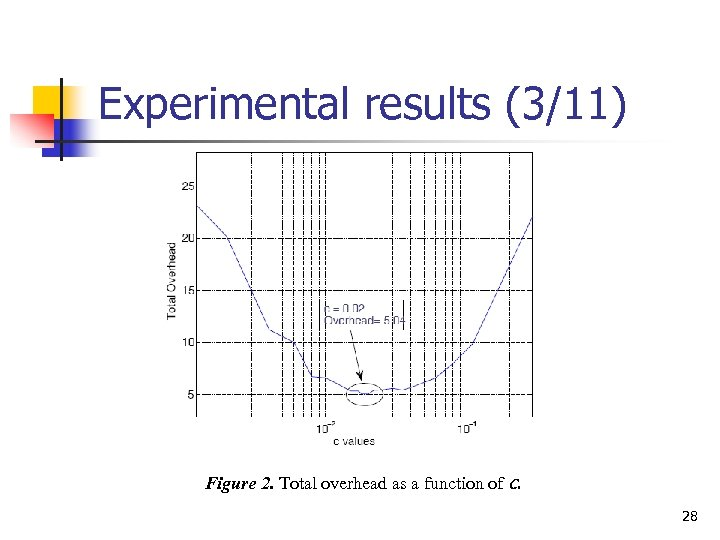Experimental results (3/11) Figure 2. Total overhead as a function of c. 28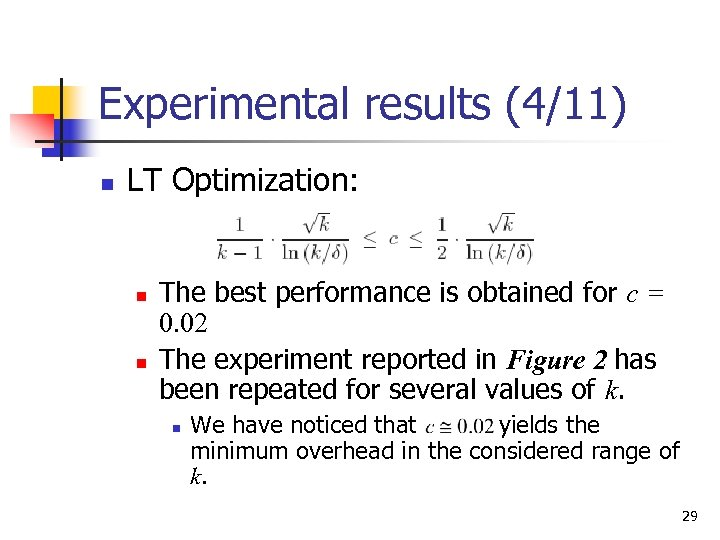Experimental results (4/11) n LT Optimization: n n The best performance is obtained for c = 0. 02 The experiment reported in Figure 2 has been repeated for several values of k. n We have noticed that yields the minimum overhead in the considered range of k. 29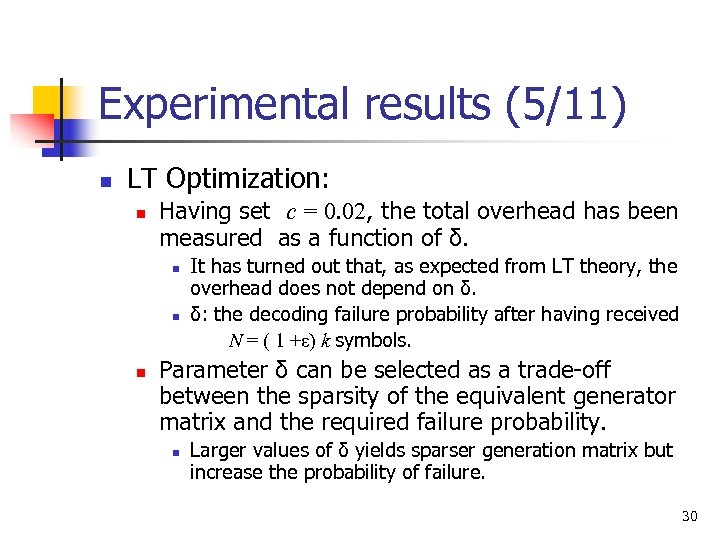Experimental results (5/11) n LT Optimization: n Having set c = 0. 02, the total overhead has been measured as a function of δ. n n n It has turned out that, as expected from LT theory, the overhead does not depend on δ. δ: the decoding failure probability after having received N = ( 1 +ε) k symbols. Parameter δ can be selected as a trade-off between the sparsity of the equivalent generator matrix and the required failure probability. n Larger values of δ yields sparser generation matrix but increase the probability of failure. 30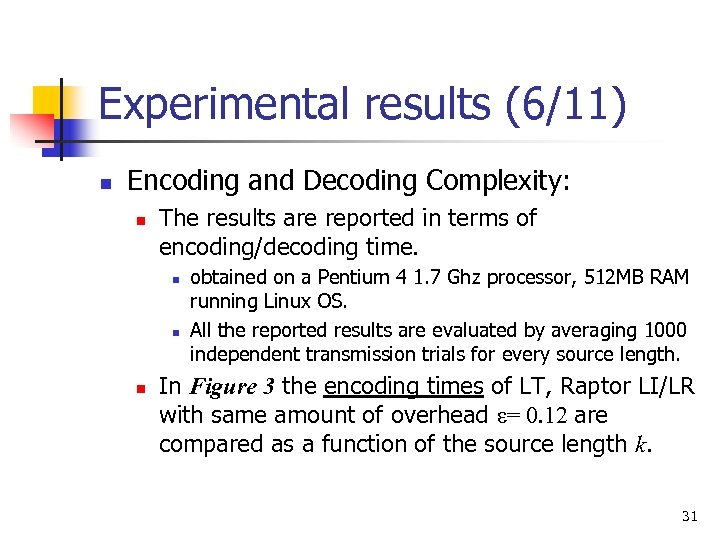Experimental results (6/11) n Encoding and Decoding Complexity: n The results are reported in terms of encoding/decoding time. n n n obtained on a Pentium 4 1. 7 Ghz processor, 512 MB RAM running Linux OS. All the reported results are evaluated by averaging 1000 independent transmission trials for every source length. In Figure 3 the encoding times of LT, Raptor LI/LR with same amount of overhead ε= 0. 12 are compared as a function of the source length k. 31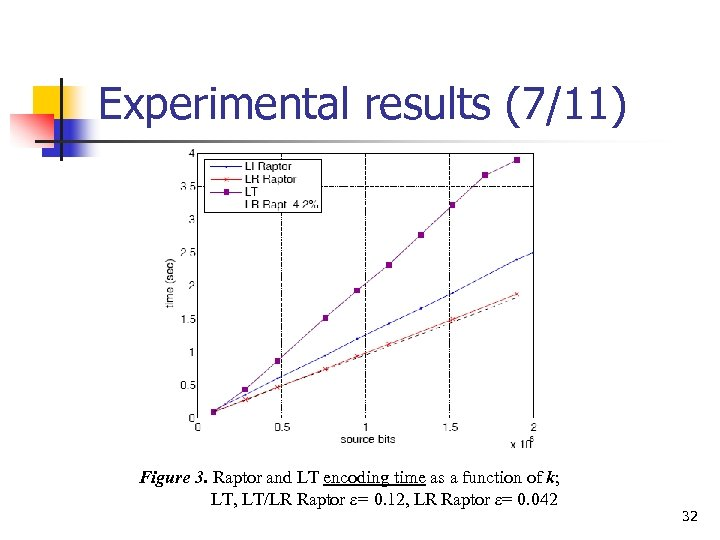Experimental results (7/11) Figure 3. Raptor and LT encoding time as a function of k; LT, LT/LR Raptor ε= 0. 12, LR Raptor ε= 0. 042 32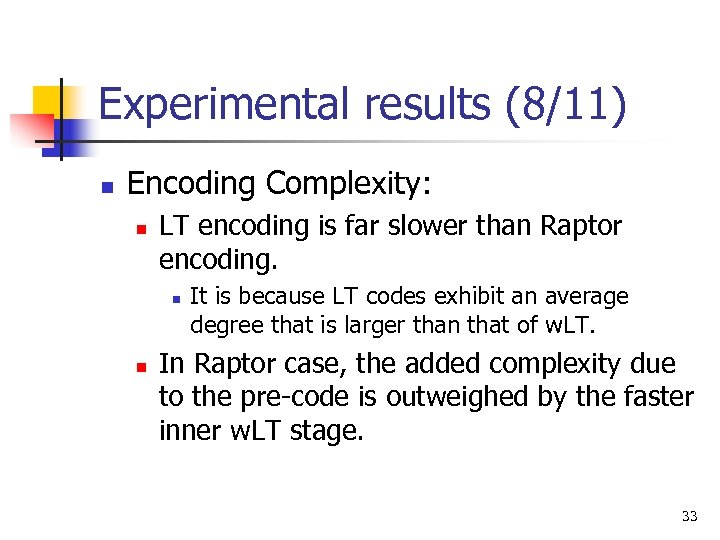Experimental results (8/11) n Encoding Complexity: n LT encoding is far slower than Raptor encoding. n n It is because LT codes exhibit an average degree that is larger than that of w. LT. In Raptor case, the added complexity due to the pre-code is outweighed by the faster inner w. LT stage. 33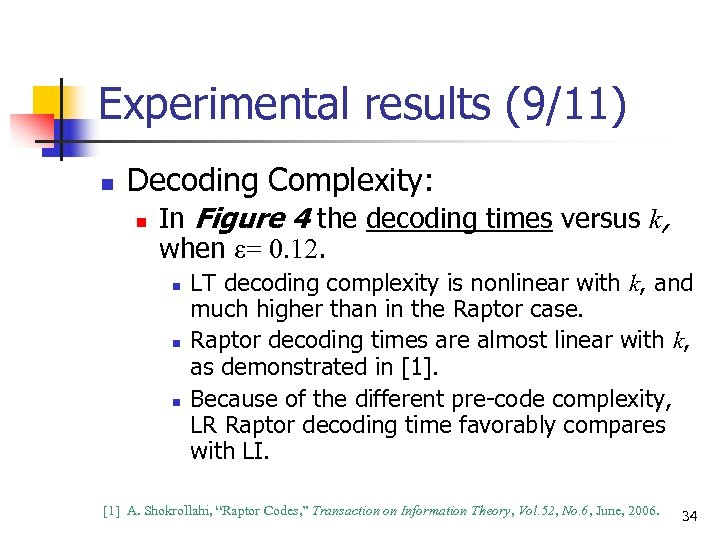Experimental results (9/11) n Decoding Complexity: n In Figure 4 the decoding times versus k, when ε= 0. 12. n n n LT decoding complexity is nonlinear with k, and much higher than in the Raptor case. Raptor decoding times are almost linear with k, as demonstrated in . Because of the different pre-code complexity, LR Raptor decoding time favorably compares with LI.  A. Shokrollahi, “Raptor Codes, ” Transaction on Information Theory, Vol. 52, No. 6, June, 2006. 34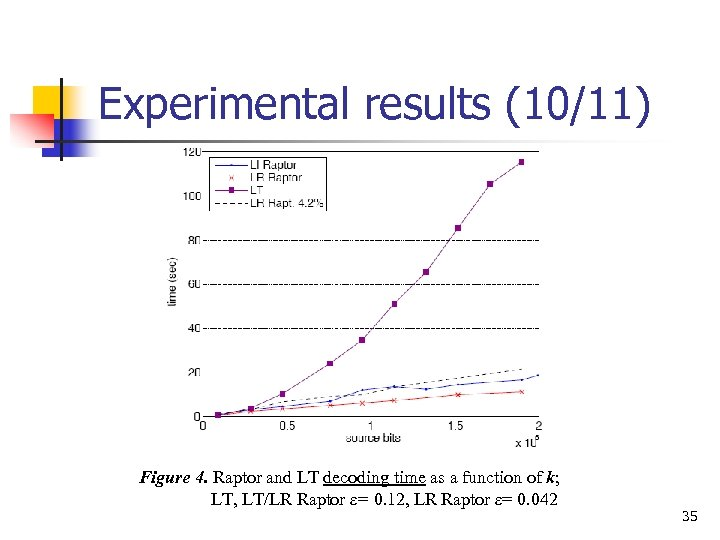Experimental results (10/11) Figure 4. Raptor and LT decoding time as a function of k; LT, LT/LR Raptor ε= 0. 12, LR Raptor ε= 0. 042 35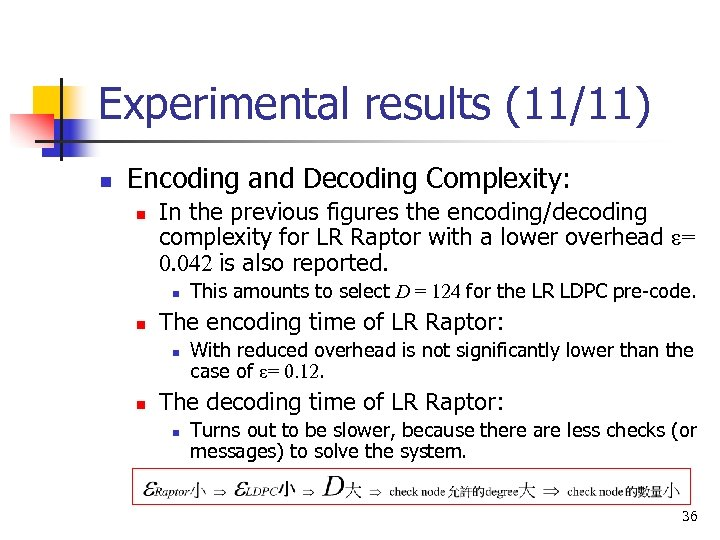Experimental results (11/11) n Encoding and Decoding Complexity: n In the previous figures the encoding/decoding complexity for LR Raptor with a lower overhead ε= 0. 042 is also reported. n n The encoding time of LR Raptor: n n This amounts to select D = 124 for the LR LDPC pre-code. With reduced overhead is not significantly lower than the case of ε= 0. 12. The decoding time of LR Raptor: n Turns out to be slower, because there are less checks (or messages) to solve the system. 36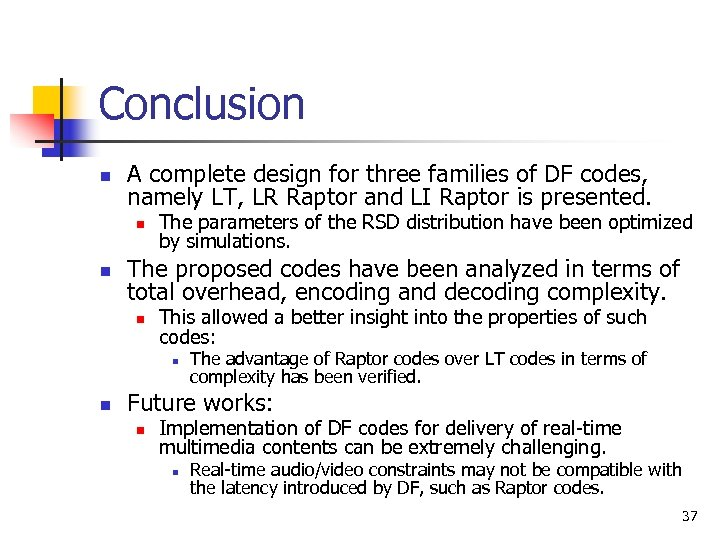Conclusion n A complete design for three families of DF codes, namely LT, LR Raptor and LI Raptor is presented. n n The parameters of the RSD distribution have been optimized by simulations. The proposed codes have been analyzed in terms of total overhead, encoding and decoding complexity. n This allowed a better insight into the properties of such codes: n n The advantage of Raptor codes over LT codes in terms of complexity has been verified. Future works: n Implementation of DF codes for delivery of real-time multimedia contents can be extremely challenging. n Real-time audio/video constraints may not be compatible with the latency introduced by DF, such as Raptor codes. 37